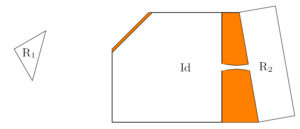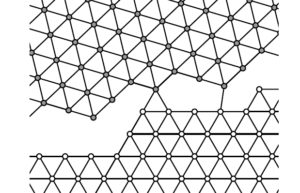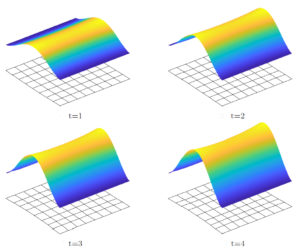Forschung

Research Interests

The general research interests of the group lie in applied analysis, more precisely in the calculus of variations and the mathematical modeling of problems in materials science. Research questions and mathematical techniques include:

• Multiscale Methods
• Gamma-convergence, relaxation, and integral representation
• Free-discontinuity problems
• Atomistic models and discrete-to-continuum limits
• Crystallization
• Geometric rigidity and linearization
• Functions of bounded variation/deformation
• Geometric measure theory
• Nonlinear evolution equations

Main research directions

The current main research directions of the group areGeometric (non-)rigidity in variable domains (Friedrich, Kreutz, Zemas)Grain boundaries in polycrystals (Friedrich, Kreutz, Schmidt)Evolution of viscoelastic von Kármán plate (Friedrich, Kruzik, Valdman)

Publications

Publications of the group, ordered by topics:

Variational models of brittle fracture

• M. Friedrich, M. Perugini, F. Solombrino: Gamma-convergence for free-discontinuity problems in linear elasticity: Homogenization and relaxation. Submitted 2020. [Preprint]
• V. Crismale, M. Friedrich, F. Solombrino: Integral representation for energies in linear elasticity with surface discontinuities. Adv. Calc. Var., to appear. [Preprint]
• M. Friedrich: Griffith energies as small strain limit of nonlinear models for nonsimple brittle materials. Mathematics in Engineering, 2 (2020), pp. 75-100. [Preprint, Article]
• M. Friedrich: A compactness result in GSBV^p and applications to Γ-convergence for free discontinuity problems. Calc. Var. PDE 58:86 (2019). [Preprint, Article]
• M. Friedrich, F. Solombrino: Quasistatic crack growth in 2d-linearized elasticity. Ann. Inst. H. Poincaré Anal. Non Linéaire 35 (2018) pp. 27-64. [Preprint, Article]
• M. Friedrich: A derivation of linearized Griffith energies from nonlinear models. Arch. Ration. Mech. Anal. 225 (2017) pp. 425-467. [Preprint, Article]

Epitaxially strained films and material voids

• M. Friedrich, L. Kreutz, K. Zemas: Geometric rigidity in variable domains and derivation of linearized models for elastic materials with free surfaces. Submitted 2021. [Preprint]
• V. Crismale, M. Friedrich: Equilibrium configurations for epitaxially strained films and material voids in three-dimensional linear elasticity. Arch. Ration. Mech. Anal. 237 (2020), pp. 1041-1098. [Preprint, Article]
• L. Kreutz, P. Piovano: Microscopic validation of a variational model of epitaxially strained crystalline film. SIAM J. Math. Anal., in press. [Preprint]

Multiphase materials

• M. Friedrich, L. Kreutz, B. Schmidt: Emergence of rigid polycrystals from atomistic systems with Heitmann-Radin sticky disk energy. Arch. Ration. Mech. Anal., to appear. [Preprint]
• E. Davoli, M. Friedrich: Two-well linearization for solid-solid phase transitions. Submitted 2020. [Preprint]
• M. Friedrich, F. Solombrino: Functionals defined on piecewise rigid functions: Integral representation and Γ-convergence. Arch. Ration. Mech. Anal. 236 (2020), pp. 1325-1387. [Preprint, Article]
• E. Davoli, M. Friedrich: Two-well rigidity and multidimensional sharp-interface limits for solid-solid phase transitions. Calc. Var. PDE 59:44 (2020). [Preprint, Article]

Foundation of the function space SBD

• M. Friedrich, M. Perugini, F. Solombrino: Lower semicontinuity for functionals defined on piecewise rigid functions and on GSBD. J. Func. Anal., to appear. [Preprint, Article]
• F. Cagnetti, A. Chambolle, M. Perugini, L. Scardia: An extension result for generalised special functions of bounded deformation. Submitted 2020. [Preprint]
• M. Friedrich: A piecewise Korn inequality in SBD and applications to embedding and density results. SIAM J. Math. Anal. 50 (2018), pp. 3842-3918. [Preprint, Article]
• M. Friedrich: On a decomposition of regular domains into John domains with uniform constants. ESAIM Control Optim. Calc. Var. 24 (2018), pp. 1541-1583. [Preprint, Article]
• M. Friedrich: A Korn-type inequality in SBD for functions with small jump sets. Math. Models Methods Appl. Sci. (M3AS) 27 (2017) pp. 2461-2484. [Preprint, Article]
• M. Friedrich, B. Schmidt: A quantitative geometric rigidity result in SBD. Preprint 2015. [Preprint]

Crystallization and lattice energies

• M. Cicalese, L. Kreutz, G. P. Leonardi: Wulff-crystals in FCC and HCP lattices. Submitted 2021. [Preprint]
• A. Chambolle, L. Kreutz: Crystallinity of the homogenized energy density of periodic lattice systems. Submitted 2021. [Preprint]
• M. Friedrich, W. Górny, U. Stefanelli: The double-bubble problem on the square lattice. Submitted 2021. [Preprint]
• (with L. Bétermin, U. Stefanelli) Lattice ground states for Embedded-Atom Models in 2D and 3D. Letters in Mathematical Physics 111: 107 (2021). [Preprint, Article]
• M. Friedrich, U. Stefanelli: Crystallization in a one-dimensional periodic landscape. J. Stat.Phys. 179 (2020), pp. 485-501. [Preprint, Article]
• M. Friedrich, L. Kreutz: Finite crystallization and Wulff shape emergence for ionic compounds in the square lattice. Nonlinearity 33 (2020), pp. 1240-1296. [Preprint, Article]
• M. Friedrich, L. Kreutz: Crystallization in the hexagonal lattice for ionic dimers. Math. Models Methods Appl. Sci. (M3AS) 29 (2019), pp. 1853-1900. [Preprint, Article]

Geometry and stability of nanostructures

• M. Friedrich, M. Seitz, U. Stefanelli: Tilings with nonflat squares: a characterization. Submitted 2021. [Preprint]
• L. Bétermin, M. Friedrich, U. Stefanelli: Angle-rigidity for Z^2 configurations. Nonlinearity, to appear
• . [Preprint]
• M. Friedrich, U. Stefanelli: Ripples in graphene: A variational approach. Comm. Math. Phys. 379 (2020), pp. 915–954. [Preprint, Article]
• M. Friedrich, E. Mainini, P. Piovano, U. Stefanelli: Characterization of optimal carbon nanotubes under stretching and validation of the Cauchy-Born rule. Arch. Ration. Mech. Anal. 231 (2019), pp. 465-517. [Preprint, Article]
• U. Ludacka, M. R. A. Monazam, C. Rentenberger, M. Friedrich, U. Stefanelli, J. C. Meyer, J. Kotakoski: In situ control over graphene ripples and strain in the electron microscope. npj 2D Materials and Applications 2: 25 (2018). [Article]
• M. Friedrich, U. Stefanelli: Graphene ground states. Zeitschrift für angewandte Mathematik und Physik, June 2018, 69:70. [Preprint, Article]
• M. Friedrich, P. Piovano, U. Stefanelli: The geometry of C60: A rigorous approach via Molecular Mechanics. SIAM J. Appl. Math. 76 (2016) pp. 2009-2029. [Preprint, Article]

Spin systems

• A. Bach, M. Cicalese, L. Kreutz, G. Orlando: The antiferromagnetic XY model on the triangular lattice: topological singularities. Submitted 2020. [Preprint]
• A. Bach, M. Cicalese, L. Kreutz, G. Orlando: The antiferromagnetic XY model on the triangular lattice: chirality transitions at the surface scaling. Submitted 2020. [Preprint]
• A. Braides, L. Kreutz: Optimal design of lattice surface energies. Calc. Var. PDE 57, 97 (2018) [Preprint, Article]
• A. Braides, L. Kreutz: Optimal bounds for periodic mixtures of nearest-neighbour ferromagnetic interactions. Rendiconti Lincei-Matematica e Applicazioni 28(1) (2017) 103–117 [Preprint, Article]

Cleavage laws in brittle crystals

• M. Friedrich, B. Schmidt: On a discrete-to-continuum convergence result for a two dimensional brittle material in the small displacement regime. Netw. Heterog. Media 10 (2015) pp. 321-342. [Preprint, Article]
• M. Friedrich, B. Schmidt: An analysis of crystal cleavage in the passage from atomistic models to continuum theory. Arch. Ration. Mech. Anal. 217 (2015) pp. 263-308. [Preprint, Article]
• M. Friedrich, B. Schmidt: An atomistic-to-continuum analysis of crystal cleavage in a two-dimensional model problem. J. Nonlin. Sci. 24 (2014) pp. 145-183. [Preprint, Article]

Nonlinear viscoelasticity

• M. Friedrich, L. Machill: Derivation of a one-dimensional von Kármán theory for viscoelastic ribbons. Submitted 2021. [Preprint]
• M. Friedrich, M. Kruzik: Derivation of von Kármán plate theory in the framework of three-dimensional viscoelasticity. Arch. Ration. Mech. Anal. 238 (2020), pp. 489-540. [Preprint, Article]
• M. Friedrich, M. Kruzik, J. Valdman: Numerical approximation of von Kármán viscoelastic plates. DCDS-S, to appear. [Preprint, Article]
• M. Friedrich, M. Kruzik: On the passage from nonlinear to linearized viscoelasticity. SIAM J. Math. Anal. 50 (2018), pp. 4426-4456. [Preprint, Article]